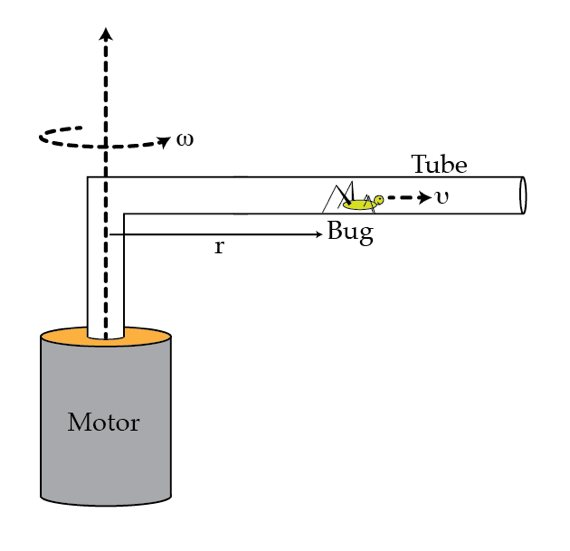# A bug in a rotating pipe

A thin massless horizontal pipe rotates about a vertical axis with angular speed $\omega$ as shown in the figure. A small bug, located at a distance $r$ from the axis, starts crawling inside the pipe at constant speed $v$ (relative to the pipe). Because of the bug, the motor must develop the power $P_{m}= C m \omega^{2}v r$ in order to keep the system rotating at constant angular speed $\omega$. Determine the numerical coefficient $C$. m is the mass of the bug.×

Problem Loading...

Note Loading...

Set Loading...